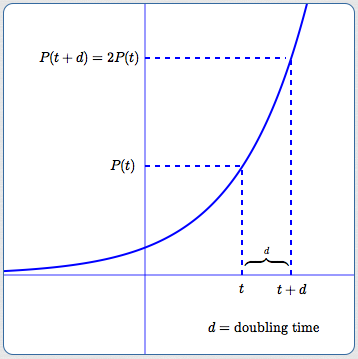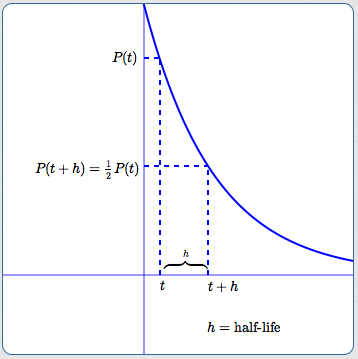﻿ Doubling Time, Half-Life

# DOUBLING TIME, HALF-LIFE

• PRACTICE (online exercises and printable worksheets)

Exponential behavior (growth/decay) is defined by:   equal changes in the input cause the output to be multiplied by a constant.

Once you've recognized exponential behavior then you can always use $\,P(t) = P_{\,0}{\text{e}}^{rt}\,$ as your model.
Here, $\,P(t)\,$ is the population at time $\,t\,$, $\,P_{\,0}\,$ is the population at time zero, and $\,r\,$ is the relative growth rate.

However, if an (equal change,constant multiplier) = $\,(\Delta t,b)\,$ pair is readily available,
then the model $\,P(t) = P_{\,0}b^{t/\Delta t}\,$ is more natural and easier to use.

A variety of problems involving exponential functions were explored in Solving Exponential Growth and Decay Problems.
This current section focuses on doubling time, half-life, and related problems.

## Doubling Time

Doubling time formula derived from $\,P(t) = P_{\,0}{\text{e}}^{rt}\,$

Doubling time $\,d\,$ depends only on the relative growth rate, $\,r\,$.
It does not depend on time!
Consequently, for exponential functions, the current population size doesn't matter;
it always takes the same amount of time for a population to double.

Let $\,P(t) = P_{\,0}{\text{e}}^{rt}\,$ for $\,r > 0\,$ (so this is exponential growth).
Let $\,d\,$ denote the doubling time, so that $\,P(t+d) = 2P(t)\,$:

 $\displaystyle\,\overbrace{\cancel{P_{\,0}}{\text{e}}^{r(t+d)}}^{P(t+d)} = \overbrace{2\cancel{P_{\,0}}{\text{e}}^{rt}}^{2P(t)}\,$ This equation must be solved for $\,d\,$. Notice that $\,P_{\,0}\,$ is immediately cancelled, so doubling time is independent of any starting population. $\displaystyle\bcancel{{\text{e}}^{rt}}{\text{e}}^{rd} = 2\bcancel{{\text{e}}^{rt}}$ Divide both sides by $\,{\text{e}}^{rt}\,$. This is the step where $\,t\,$ disappears! No matter what the size of the current population, it takes the same time to double. $\ln {\text{e}}^{rd} = \ln 2$ ‘Undo’ the exponent with a logarithm. Any log will work; the natural log is chosen. $rd = \ln 2$ Use a property of logs to bring down the exponent. $\displaystyle d = \frac{\ln 2}{r}$ Solve for $\,d\,$ by dividing both sides by $\,r\,$.The doubling time $\,d\,\,$ for the exponential model $\,P(t) = P_{\,0}{\text{e}}^{rt}\,$ ($\,r > 0\,$) is:

$\displaystyle d = \frac{\ln 2}{r}$
Doubling time formula derived from $\,P(t) = P_{\,0}b^{t/\Delta t}\,$

As above, doubling time does not depend on time!
It depends only on the (equal change,constant multiplier) = $\,(\Delta t,b)\,$ pair.

Let $\,P(t) = P_{\,0}b^{t/\Delta t}\,$ for $\,b > 1\,$ (so this is exponential growth).
Let $\,d\,$ denote the doubling time, so that $\,P(t+d) = 2P(t)\,$:

$\,\overbrace{\cancel{P_{\,0}}b^{(t+d)/\Delta t}}^{P(t+d)} = \overbrace{2\cancel{P_{\,0}}b^{t/\Delta t}}^{2P(t)}\,$

$\,\cancel{b^{t/\Delta t}} b^{d/\Delta t} = 2\cancel{b^{t/\Delta t}}\,$     (Note that $\,t\,$ disappears at this step!)

$b^{d/\Delta t} = 2$

$\displaystyle\ln b^{d/\Delta t} = \ln 2$

$\displaystyle\frac{d}{\Delta t}\ln b = \ln 2$

$\displaystyle d = \frac{\ln 2}{\ln b}\cdot \Delta t$

The doubling time $\,d\,\,$ for the exponential model $\,P(t) = P_{\,0}b^{t/\Delta t}\,$ ($\,b > 1\,$) is:

$\displaystyle d = \frac{\ln 2}{\ln b}\cdot \Delta t$
What is the relative growth rate for $\,P_{\,0}b^{t/\Delta t}\,$?

Putting $\,P(t) = P_{\,0}b^{t/\Delta t}\,$ in the form $\,P(t) = P_{\,0}{\text{e}}^{rt}\,$ yields:
$\displaystyle P_{\,0}b^{t/\Delta t} = P_{\,0}{\text{e}}^{\ln b^{t/\Delta t}} = P_{\,0}{\text{e}}^{(t/\Delta t)\ln b} = P_{\,0}{\text{e}}^{t\overbrace{(\ln b)/\Delta t}^{r}}$

Thus, the relative growth rate for $\,P_{\,0}b^{t/\Delta t}\,$ is $\displaystyle\,r = \frac{\ln b}{\Delta t}\,$.

Compatibility of the two doubling time formulas

Using the fact that $\displaystyle\,r = \frac{\ln b}{\Delta t}\,$, we have:
$\displaystyle d = \frac{\ln 2}{\ln b}\cdot \Delta t = \frac{\ln 2}{(\ln b/\Delta t)} = \frac{\ln 2}{r}$

## Half-Life

Half-life is the time it takes for a current amount to be cut in half.

 Half-life formula derived from $\,P(t) = P_{\,0}{\text{e}}^{rt}\,$ Again, half-life depends only on the relative growth rate, $\,r\,$. It does not depend on time! Let $\,P(t) = P_{\,0}{\text{e}}^{rt}\,$ for $\,r < 0\,$ (so this is exponential decay). Let $\,h\,$ denote the half-life, so $\,P(t+h) = \frac 12P(t)\,$: $\displaystyle\,\overbrace{\cancel{P_{\,0}}{\text{e}}^{r(t+h)}}^{P(t+h)} = \overbrace{\frac 12\cancel{P_{\,0}}{\text{e}}^{rt}}^{\frac 12P(t)}\,$ $\displaystyle\bcancel{{\text{e}}^{rt}}{\text{e}}^{rh} = \frac 12\bcancel{{\text{e}}^{rt}}$   (Note that $\,t\,$ disappears at this step!) $\ln {\text{e}}^{rh} = \ln \frac 12 = \ln 0.5$ $rh = \ln 0.5$ $\displaystyle h = \frac{\ln 0.5}{r} = \frac{\ln 2^{-1}}{r} = -\frac{\ln 2}{r}$ Note that since $\,r < 0\,$ for exponential decay, we have $\displaystyle \,h = -\frac{\ln 2}{r} > 0\,$.The half-life $\,h\,\,$ for the exponential model $\,P(t) = P_{\,0}{\text{e}}^{rt}\,$ ($\,r < 0\,$) is:

$\displaystyle h = \frac{\ln 0.5}{r} = -\frac{\ln 2}{r}$
Half-life formula derived from $\,P(t) = P_{\,0}b^{t/\Delta t}\,$

As above, half-life does not depend on time!
It depends only on the (equal change,constant multiplier) = $\,(\Delta t,b)\,$ pair.

Let $\,P(t) = P_{\,0}b^{t/\Delta t}\,$ for $\,b < 1\,$ (so this is exponential decay).
Let $\,h\,$ denote the half-life, so that $\,P(t+h) = \frac 12P(t)\,$:

$\,\overbrace{\cancel{P_{\,0}}b^{(t+h)/\Delta t}}^{P(t+h)} = \overbrace{\frac 12\cancel{P_{\,0}}b^{t/\Delta t}}^{\frac 12P(t)}\,$

$\,\cancel{b^{t/\Delta t}} b^{h/\Delta t} = \frac 12\cancel{b^{t/\Delta t}}\,$     (Note that $\,t\,$ disappears at this step!)

$b^{h/\Delta t} = \frac 12 = 0.5$

$\displaystyle\ln b^{h/\Delta t} = \ln 0.5$

$\displaystyle\frac{h}{\Delta t}\ln b = \ln 0.5$

$\displaystyle h = \frac{\ln 0.5}{\ln b}\cdot \Delta t = \frac{\ln 2^{-1}}{\ln b}\cdot \Delta t = -\frac{\ln 2}{\ln b}\cdot \Delta t$

Note that since $\,b < 1\,$ for exponential decay, we have $\,\ln b < 0\,$, and thus $\displaystyle h = -\frac{\ln 2}{\ln b}\cdot \Delta t > 0\,$.

The half-life $\,h\,\,$ for the exponential model $\,P(t) = P_{\,0}b^{t/\Delta t}\,$ ($\,b < 1\,$) is:

$\displaystyle h = \frac{\ln 0.5}{\ln b}\cdot \Delta t = -\frac{\ln 2}{\ln b}\cdot \Delta t$

## Generalization: How long does it take for a population to go from $\,P(t)\,$ to $\,kP(t)\,$?

Let $\,g\,$ be the time it takes to go from $\,P(t)\,$ to $\,kP(t)\,$ for any $\,k > 0\,$.
The derivation is identical to those above, hence is greatly abbreviated here:
 $$\begin{gather} P(t) = P_{\,0}{\text{e}}^{rt}\cr\cr P(t+g) = kP(t)\cr\cr P_{\,0}{\text{e}}^{r(t+g)} = kP_{\,0}{\text{e}}^{rt}\cr\cr {\text{e}}^{rg} = k\cr\cr g = \frac{\ln k}{r} \end{gather}$$ $$\begin{gather} P(t) = P_{\,0}b^{t/\Delta t}\cr\cr P(t+g) = kP(t)\cr\cr P_{\,0}b^{(t+g)/\Delta t} = kP_{\,0}b^{t/\Delta t}\cr\cr b^{g/\Delta t} = k\cr\cr \frac{g}{\Delta t}\ln b = \ln k\cr\cr g = \frac{\ln k}{\ln b}\cdot \Delta t \end{gather}$$

Master the ideas from this section